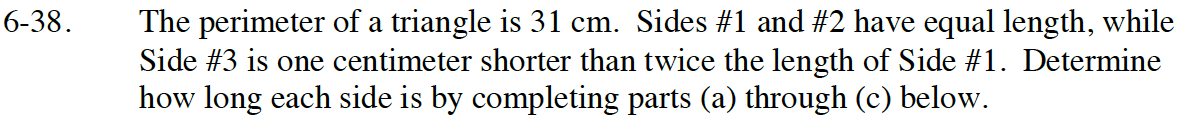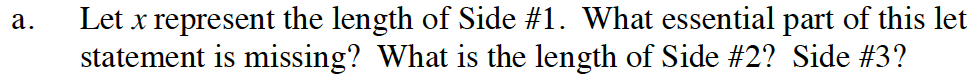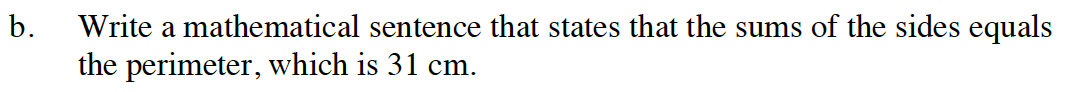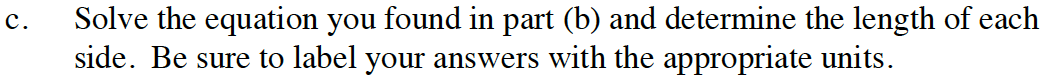Home > GB8I > Chapter cc46 > Lesson cc46.1.4 > Problem6-38

6-38.
1. The perimeter of a triangle is 31 cm. Sides #1 and #2 have equal length, while Side #3 is one centimeter shorter than twice the length of Side #1. Determine how long each side is by completing parts (a) through (c) below. Homework Help ✎

1. Let x represent the length of Side #1. What essential part of this let statement is missing? What is the length of Side #2? Side #3?

2. Write a mathematical sentence that states that the sums of the sides equals the perimeter, which is 31 cm.

3. Solve the equation you found in part (b) and determine the length of each side. Be sure to label your answers with the appropriate units.According to the problem, side #2 is the same length as side #1.
Write an expression representing side #3 using x

Side #2 = x
Side #3 = 2x - 1Side #1 + Side #2 + Side #3 = 31x + x + 2x − 1 = 31

Once you've found the value of x, find the length of the sides including the units.